# Artificial Intelligence Lecture 8 Cut Predicates The cut

• Slides: 35
Download presentationArtificial Intelligence Lecture 8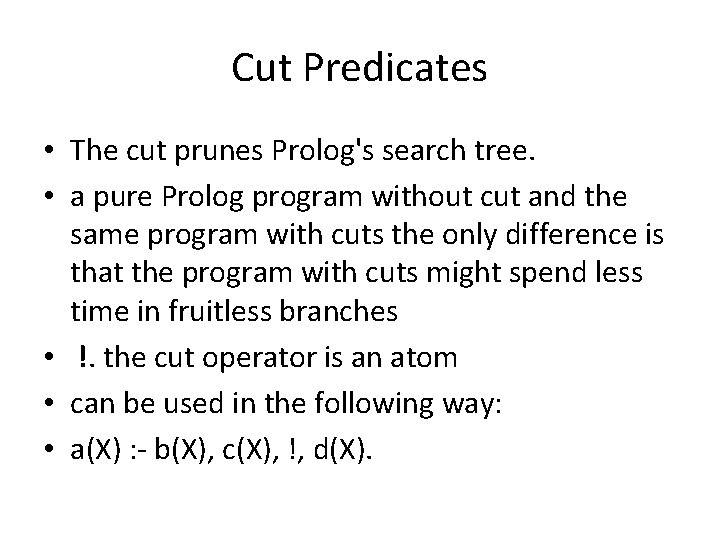Cut Predicates • The cut prunes Prolog's search tree. • a pure Prolog program without cut and the same program with cuts the only difference is that the program with cuts might spend less time in fruitless branches • !. the cut operator is an atom • can be used in the following way: • a(X) : - b(X), c(X), !, d(X).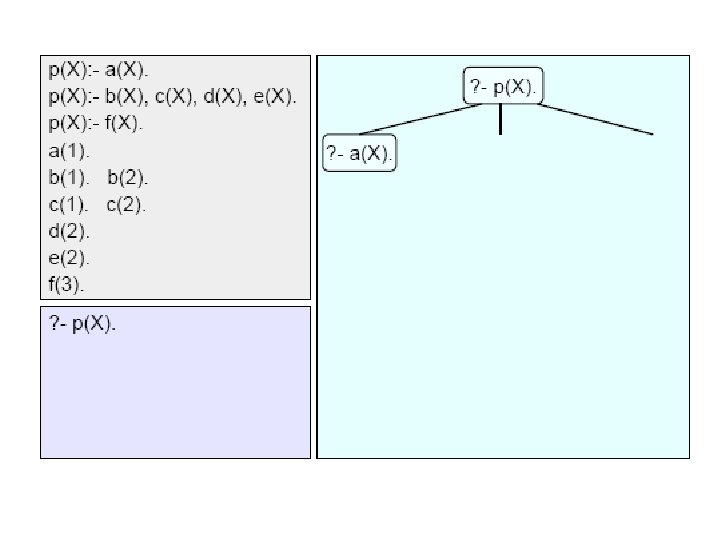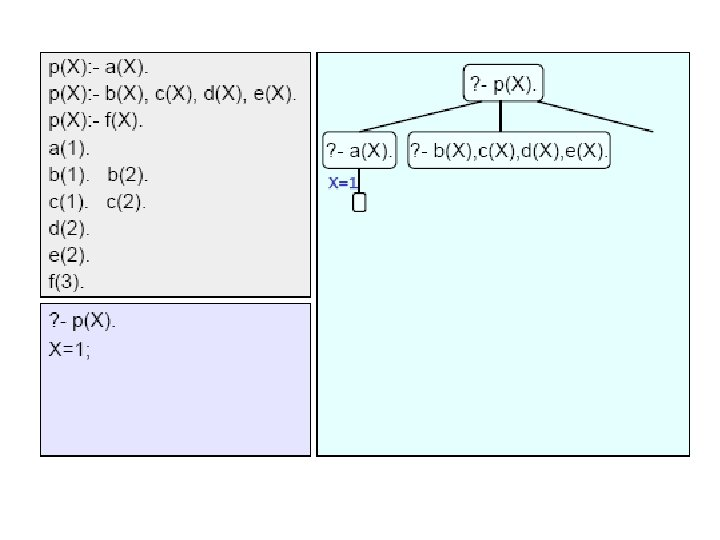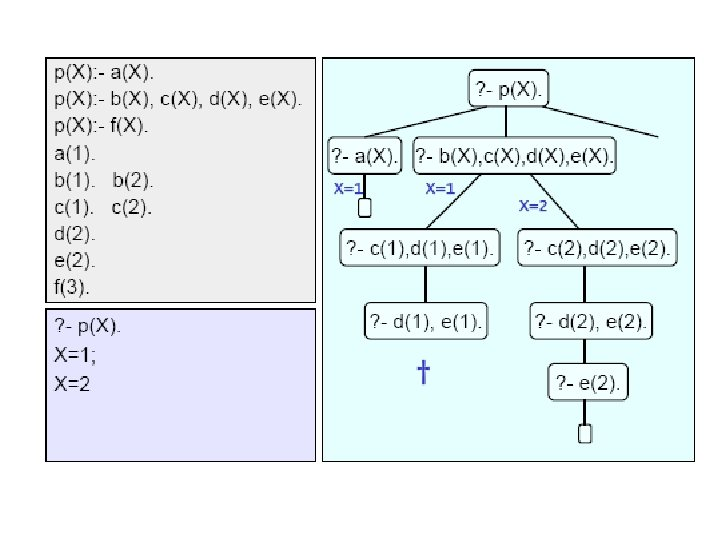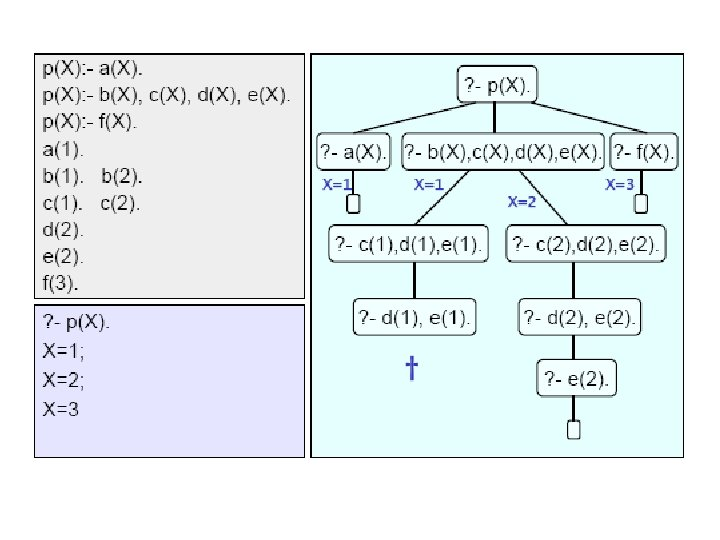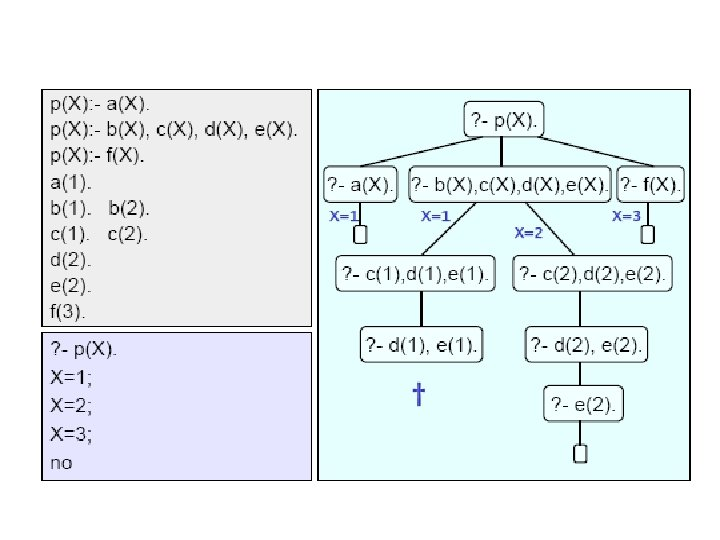Adding a cut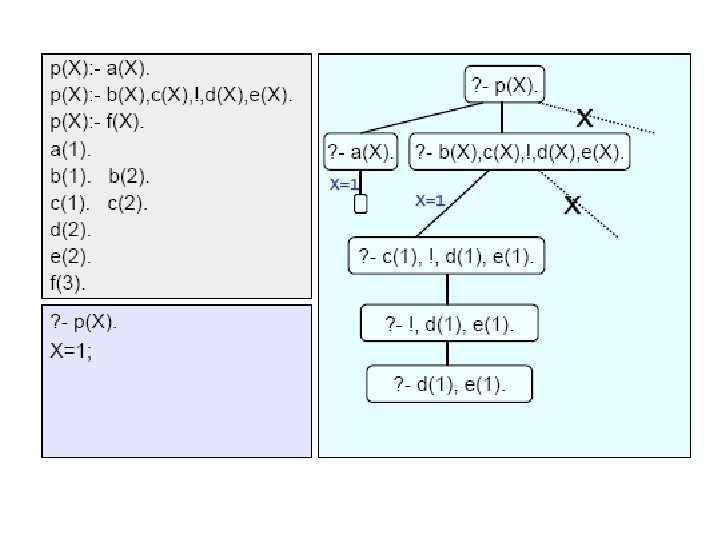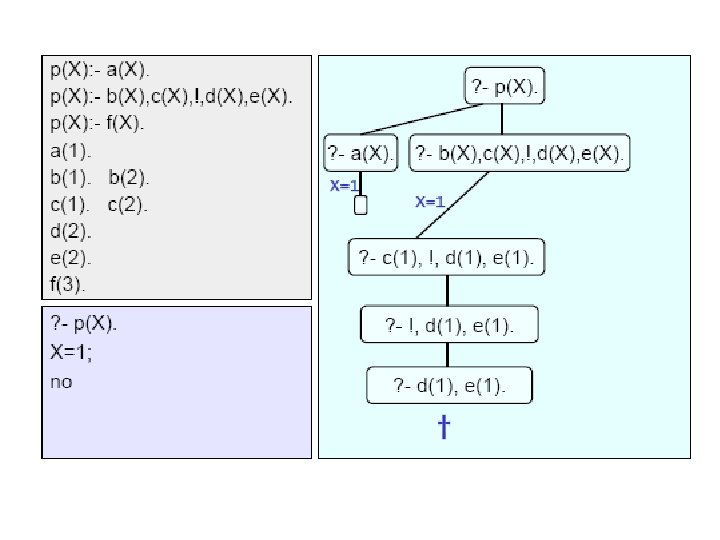Recursion • Predicates can be deﬁned recursively. Roughly speaking, a predicate is recursively deﬁned if one or more rules in its deﬁnition refers to itself. • Recursion is usually used in two situations: • when relations are described with the help of the relations themselves • when compound objects are a part of other compound objects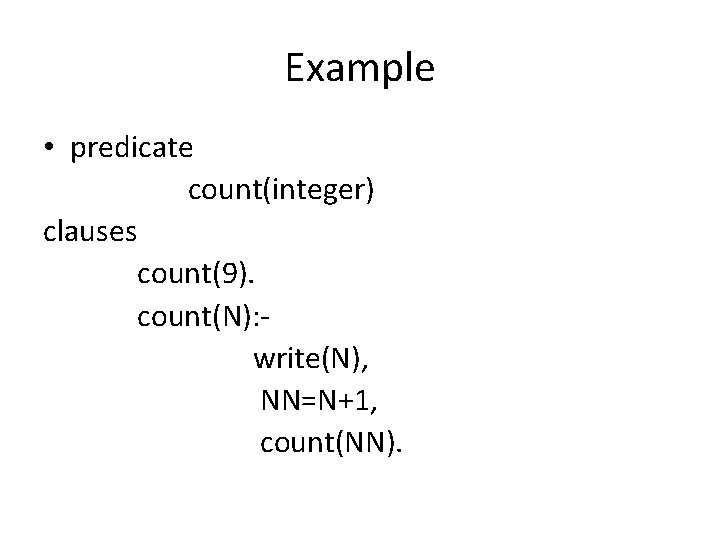Example • predicate count(integer) clauses count(9). count(N): write(N), NN=N+1, count(NN).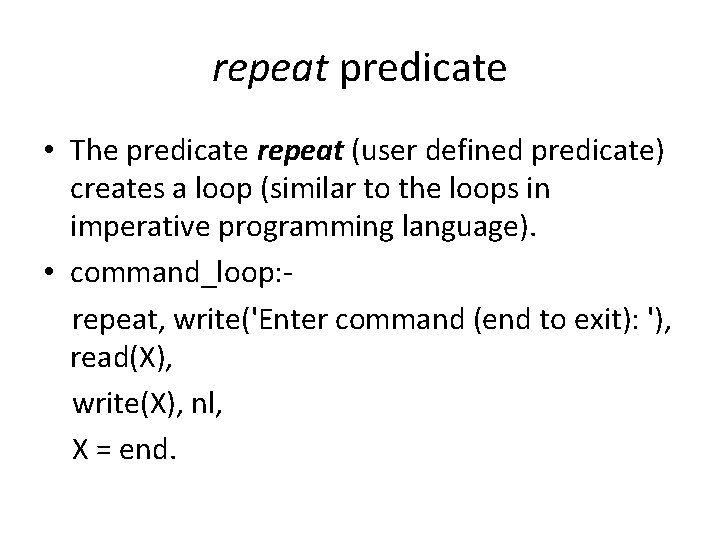repeat predicate • The predicate repeat (user defined predicate) creates a loop (similar to the loops in imperative programming language). • command_loop: repeat, write('Enter command (end to exit): '), read(X), write(X), nl, X = end.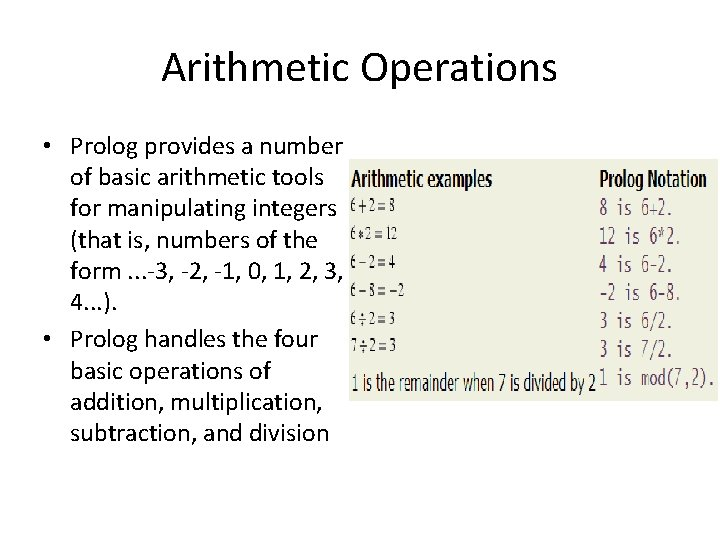Arithmetic Operations • Prolog provides a number of basic arithmetic tools for manipulating integers (that is, numbers of the form. . . -3, -2, -1, 0, 1, 2, 3, 4. . . ). • Prolog handles the four basic operations of addition, multiplication, subtraction, and divisionOperators that compare Integers• A simple example of their use would be the following two predicates: • positive(N) : - N>0. • non_zero(N) : - N<0; N>0.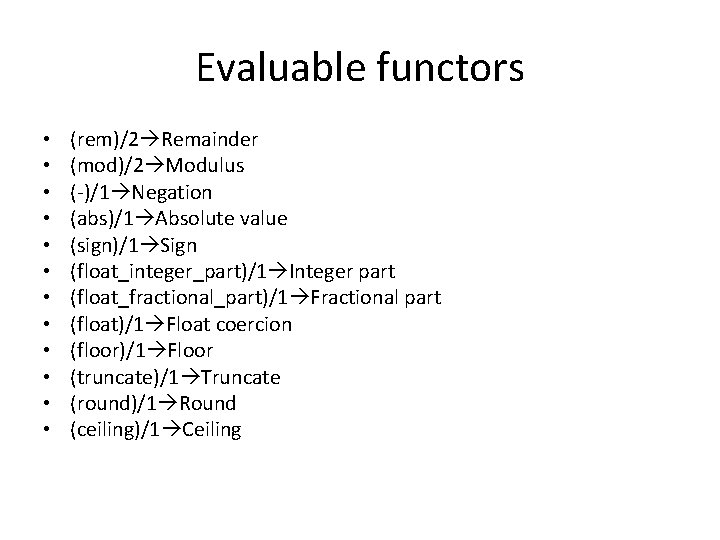Evaluable functors • • • (rem)/2 Remainder (mod)/2 Modulus (-)/1 Negation (abs)/1 Absolute value (sign)/1 Sign (float_integer_part)/1 Integer part (float_fractional_part)/1 Fractional part (float)/1 Float coercion (floor)/1 Floor (truncate)/1 Truncate (round)/1 Round (ceiling)/1 Ceiling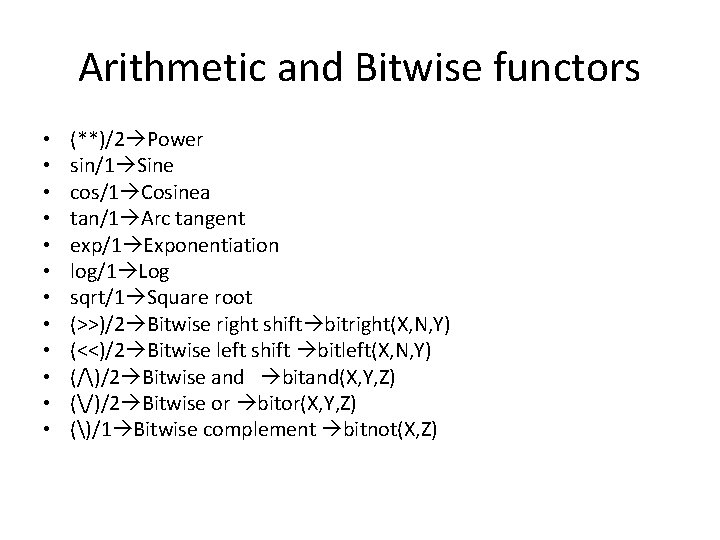Arithmetic and Bitwise functors • • • (**)/2 Power sin/1 Sine cos/1 Cosinea tan/1 Arc tangent exp/1 Exponentiation log/1 Log sqrt/1 Square root (>>)/2 Bitwise right shift bitright(X, N, Y) (<<)/2 Bitwise left shift bitleft(X, N, Y) (/)/2 Bitwise and bitand(X, Y, Z) (/)/2 Bitwise or bitor(X, Y, Z) ()/1 Bitwise complement bitnot(X, Z)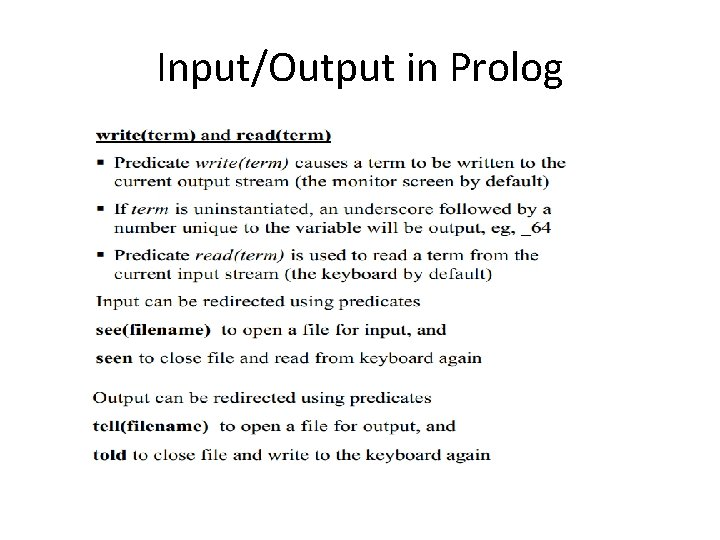Input/Output in Prolog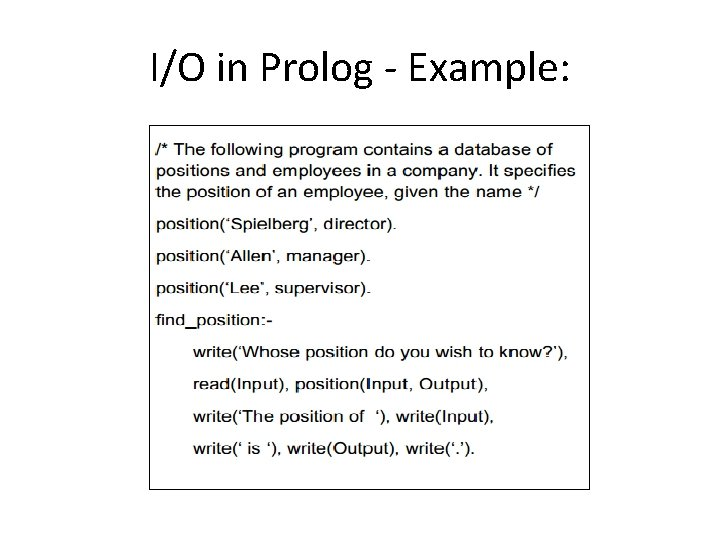I/O in Prolog - Example: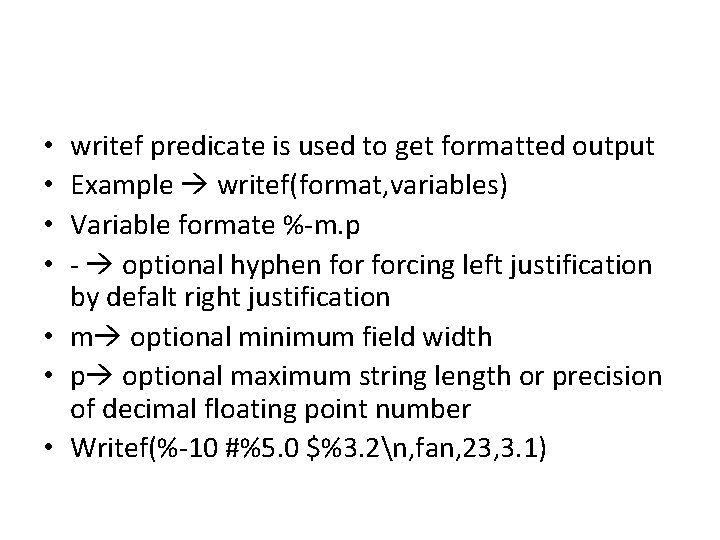writef predicate is used to get formatted output Example writef(format, variables) Variable formate %-m. p - optional hyphen forcing left justification by defalt right justification • m optional minimum field width • p optional maximum string length or precision of decimal floating point number • Writef(%-10 #%5. 0 \$%3. 2n, fan, 23, 3. 1) • •Input predicates • • • Readln read string or symbol Readchar / inkey read single character Readint read integer Readreal read real Keypressed a key is pressed or not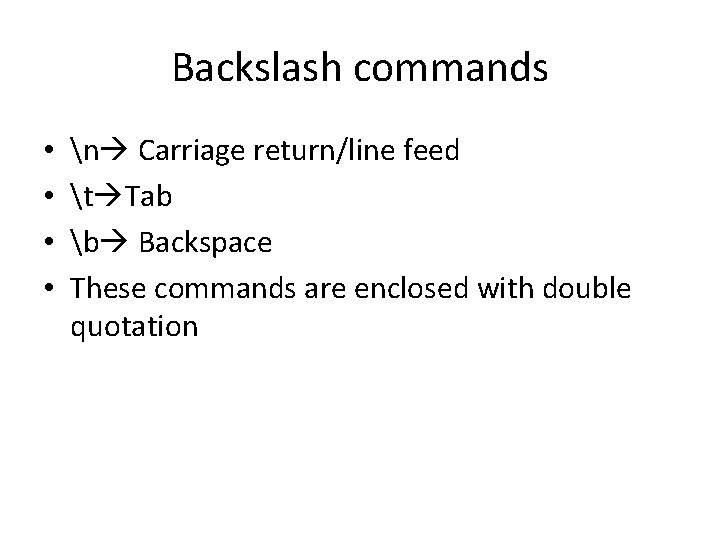Backslash commands • • n Carriage return/line feed t Tab b Backspace These commands are enclosed with double quotation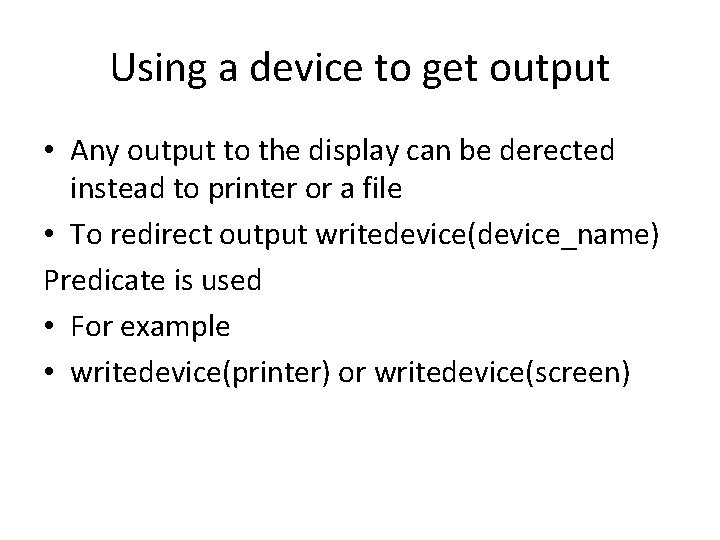Using a device to get output • Any output to the display can be derected instead to printer or a file • To redirect output writedevice(device_name) Predicate is used • For example • writedevice(printer) or writedevice(screen)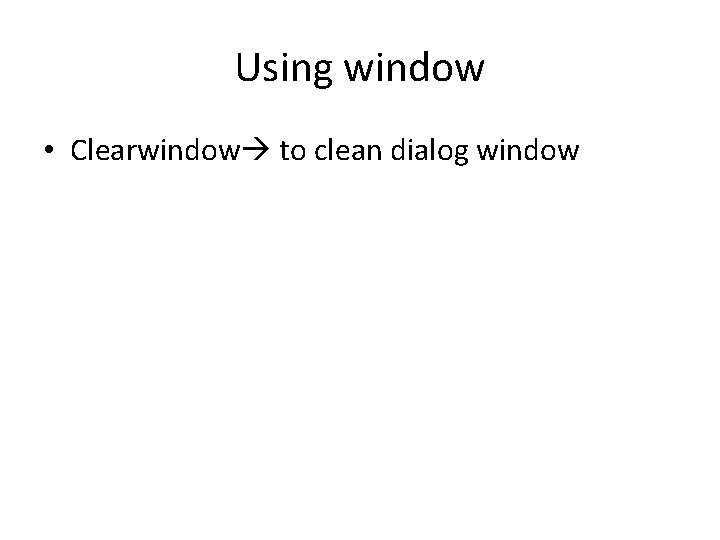Using window • Clearwindow to clean dialog windowDatabase in prolog • The database is a non-logical extension to Prolog. • The core philosophy of Prolog is that is searches for a set of variable bindings that makes a query true.• Prolog has four database manipulation commands: • assert, retract, asserta, and assertz.assertz and asserta • asserta(fact) predicate stores a fact at the beginning of the database • assertz(fact) Places asserted material at the end of the database • All variables are bound before the database predicate is invoked • assert commands always succeedExample • • Consider an empty database. If we now give the command: listing. we simply get a yes; the listing (of course) is empty. we now give this command: assert(happy(mia)). If we now give the command: listing. we get the listing: happy(mia). • • • we then made four more assert commands: assert(happy(vincent)). yes assert(happy(marcellus)). yes assert(happy(butch)). yes assert(happy(vincent)). yes. Suppose we then ask for a listing: listing. happy(mia). happy(vincent). happy(marcellus). happy(butch). happy(vincent). yes• we have only asserted facts into the database, but we can assert new rules as well. Suppose we want to assert the rule that everyone who is happy is naive. That is, suppose we want to assert that: • naive(X) : - happy(X). We can do this as follows: • assert( (naive(X) : happy(X)) ). • If we now ask for a listing we get: • happy(mia). happy(vincent). happy(marcellus). happy(butch). happy(vincent). naive(A) : happy(A).retract • Used to remove things form the database when we no longer need them • retract(happy(marcellus )). • then list the database we get: • happy(mia). happy(vincent). happy(butch). happy(vincent). naive(A) : happy(A).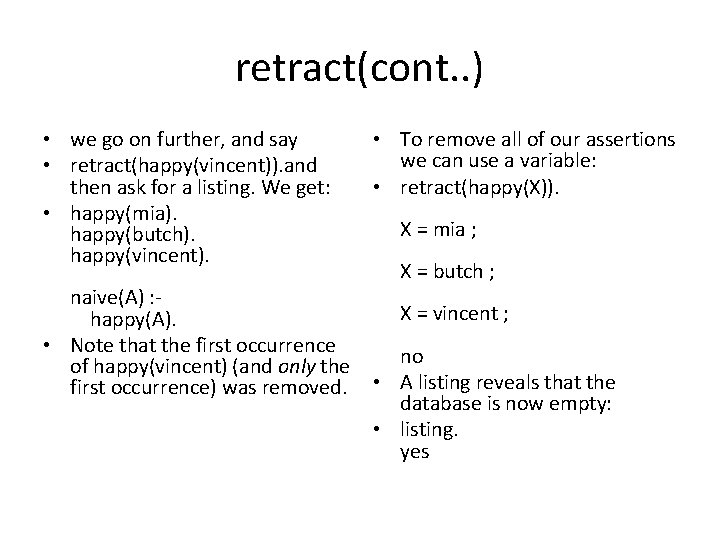retract(cont. . ) • we go on further, and say • To remove all of our assertions we can use a variable: • retract(happy(vincent)). and then ask for a listing. We get: • retract(happy(X)). • happy(mia). X = mia ; happy(butch). happy(vincent). X = butch ; naive(A) : X = vincent ; happy(A). • Note that the first occurrence no of happy(vincent) (and only the first occurrence) was removed. • A listing reveals that the database is now empty: • listing. yes